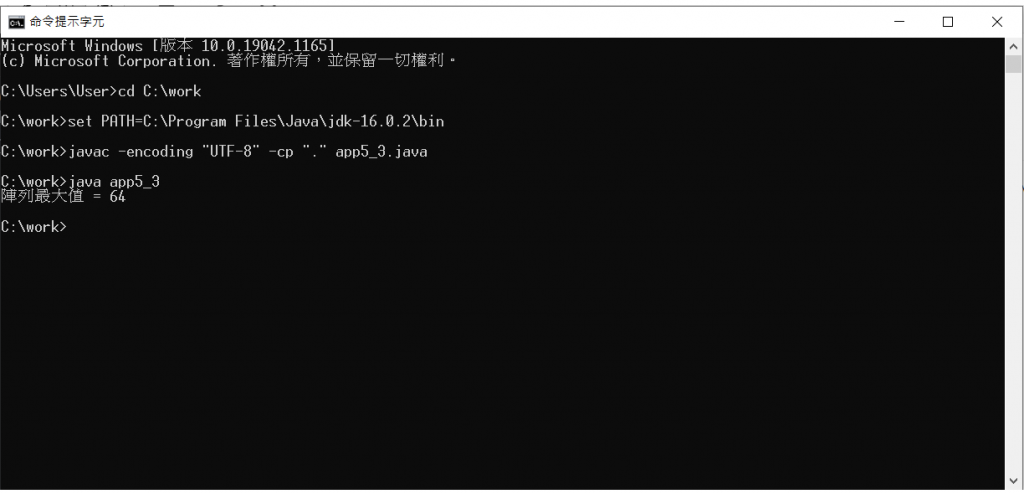#DAY 18
0

## [iT鐵人賽Day18]JAVA的函數(下篇)

``````import java.util.*;
import static java.lang.System.*;
public class app5_2
{
public static void main(String[] args) {
double num;
num = show_length(3,4);
System.out.println("對角線長度 = " + num);
}
public static double show_length(int m,int n) {
return Math.sqrt(m*m+n*n);
}
}
````````````import java.util.*;
import static java.lang.System.*;
public class app5_3
{
public static void main(String[] args) {
int a [] = {9,15,64,25,18,54};
largest(a);
}
public static void largest(int arr[]) {
int max = arr;
for(int i = 0;i < arr.length;i++)
if(max < arr[i])
max = arr[i];
System.out.println("陣列最大值 = " + max);
}
}
``````